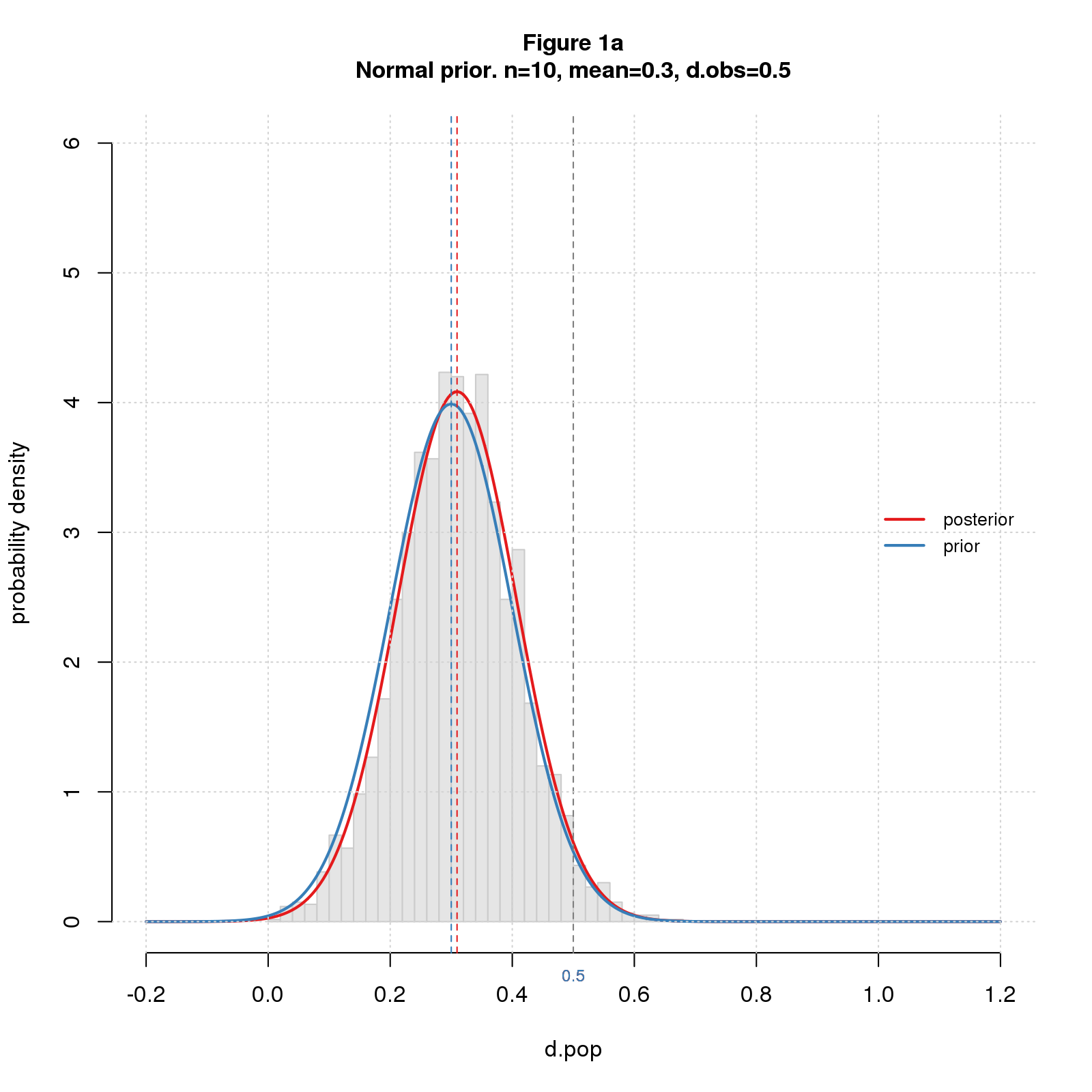What’s the true effect size? That’s my bottom line question when doing a study or reading a paper. I don’t expect an exact answer, of course. What I want is a probability distribution telling where the true effect size probably lies. I used to think confidence intervals answered this question, but they don’t except under artificial conditions. A better answer comes from Bayes’s formula. But beware of the devil in the priors.

Confidence intervals, like other standard methods such as the t-test, imagine we’re repeating a study an infinite number of times, drawing a different sample each time from the same population. That seems unnatural for basic, exploratory research, where the usual practice is to run a study once (or maybe twice for confirmation).

As I looked for a general way to estimate true effect size from studies done once, I fell into Bayesian analysis. Much to my surprise, this proved to be simple and intuitive. The code for the core Bayesian analysis (available here) is simple, too: just a few lines of R. The main drawback is the answer depends on your prior expectation. Upon reflection, this drawback may really be a strength, because it forces you to articulate key assumptions.

Being a programmer, I always start with simulation when learning a new statistical method. I model the scenario as a two stage random process. The first stage selects a population (aka “true”) effect size, \(d_{pop}\), from a distribution; the second carries out a study with that population effect size yielding an observed effect size, \(d_{obs}\). The studies are simple two group difference-of-mean studies with equal sample size and standard deviation, and the effect size statistic is standardized difference (aka Cohen’s d). I record \(d_{pop}\) and \(d_{obs}\) from each simulation producing a table showing which \(d_{pop}\)s give rise to which \(d_{obs}\)s. Then I pick a target value for \(d_{obs}\), say \(0.5\), and limit the table to rows where \(d_{obs}\) is near \(0.5\). The distribution of \(d_{pop}\) from this subset is the answer to my question. In Bayesian-speak, the first-stage distribution is the prior, and the final distribution is the posterior.

Now for the cool bit. The Bayesian approach lets us pick a prior that represents our assumptions about the distribution of effect sizes in our research field. From what I read in the blogosphere, the typical population effect size in social science research is \(0.3\). I model this as a normal distribution with \(mean=0.3\) and small standard deviation, \(0.1\). I also do simlulations with a bigger prior, \(mean=0.7\), to illustrate the impact of the choice.

Figures 1a-d show the results for small and large samples (\(n=10\) or \(200\)) and small and big priors for \(d_{obs}=0.5\). Each figure shows a histogram of simulated data, the prior and posterior distributions (blue and red curves), the medians of the two distributions (blue and red dashed vertical lines), and \(d_{obs}\) (gray dashed vertical line).